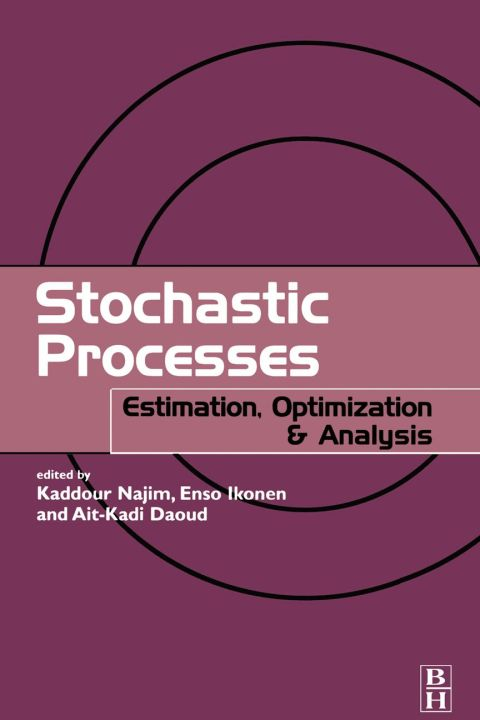• Edition
• PublishedJuly 2004
• PublisherElsevier S & T
• ISBN9781903996553
A ‘stochastic’ process is a ‘random’ or ‘conjectural’ process, and this book is concerned with applied probability and statistics. Whilst maintaining the mathematical rigour this subject requires, it addresses topics of interest to engineers, such as problems in modelling, control, reliability maintenance, data analysis and engineering involvement with insurance.

This book deals with the tools and techniques used in the stochastic process – estimation, optimisation and recursive logarithms – in a form accessible to engineers and which can also be applied to Matlab.

Amongst the themes covered in the chapters are mathematical expectation arising from increasing information patterns, the estimation of probability distribution, the treatment of distribution of real random phenomena (in engineering, economics, biology and medicine etc), and expectation maximisation. The latter part of the book considers optimization algorithms, which can be used, for example, to help in the better utilization of resources, and stochastic approximation algorithms, which can provide prototype models in many practical applications.

* An engineering approach to applied probabilities and statistics
* Presents examples related to practical engineering applications, such as reliability, randomness and use of resources
* Readers with varying interests and mathematical backgrounds will find this book accessible

# Stochastic Processes: Estimation, Optimisation and Analysis

##### Get it instantly

Zookal account needed

\$269.02 \$275.00 Save \$5.98
• Edition
• PublishedJuly 2004
• PublisherElsevier S & T
• ISBN9781903996553
A ‘stochastic’ process is a ‘random’ or ‘conjectural’ process, and this book is concerned with applied probability and statistics. Whilst maintaining the mathematical rigour this subject requires, it addresses topics of interest to engineers, such as problems in modelling, control, reliability maintenance, data analysis and engineering involvement with insurance.

This book deals with the tools and techniques used in the stochastic process – estimation, optimisation and recursive logarithms – in a form accessible to engineers and which can also be applied to Matlab.

Amongst the themes covered in the chapters are mathematical expectation arising from increasing information patterns, the estimation of probability distribution, the treatment of distribution of real random phenomena (in engineering, economics, biology and medicine etc), and expectation maximisation. The latter part of the book considers optimization algorithms, which can be used, for example, to help in the better utilization of resources, and stochastic approximation algorithms, which can provide prototype models in many practical applications.

* An engineering approach to applied probabilities and statistics
* Presents examples related to practical engineering applications, such as reliability, randomness and use of resources
* Readers with varying interests and mathematical backgrounds will find this book accessible